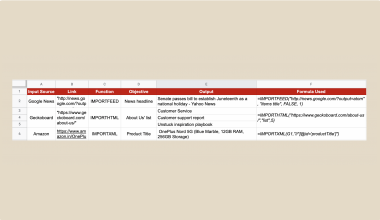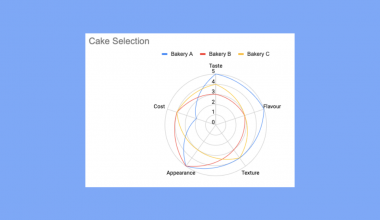# How To Use IMLOG Function in Google Sheets

The IMLOG function in Google Sheets is useful when you need to compute the logarithm of a complex number for a given base.

Since complex numbers are often read as text by Google Sheets, we require a special formula to deal with these values. Using the IMLOG function allows us to calculate logarithms when complex numbers are provided.

The rules for using the `IMLOG` function in Google Sheets are as follows:

• The function requires two arguments. The input value and the base to use when calculating the logarithm
• The function then outputs the result of the logarithmic calculation
• The base must be a positive real number

Let’s take a look at a quick example!

In this particular example, we are given a column of complex numbers and another column of corresponding bases. We would like to know the value of the logarithm of a given complex number x using the given base y.

With the `IMLOG` function in Google Sheets, it becomes straightforward enough to make this calculation.

Do note that Google Sheets also has several other functions that work similarly.  The functions `IMLOG2` and `IMLOG10` are equivalent to using `IMLOG` with the base argument of 2 and 10, respectively. `IMLOG` is also practically the same as the `LOG` function for all real number values greater than zero.

Now that you know when we can use our `IMLOG` function, let’s learn how to write it ourselves in Google Sheets and later test out the function with actual complex numbers.

## The Anatomy of the IMLOG Function

So the syntax (the way we write) of the `IMLOG` function is as follows:

```=IMLOG(value, base)
```

Let’s dissect this thing and understand what each of these terms means:

• = the equal sign is how we start any function in Google Sheets.
• IMLOG() is our `IMLOG` function. It computes the logarithm of a given complex number using a given base.
• value refers to the input value of the logarithm function.
• base refers to the value of the base used when calculating the logarithm.

## A Real Example of Using IMLOG Function

Let’s look into an example of the `IMLOG` function being used in a Google Sheet spreadsheet.

In the table below, we have our given values and bases. Using the `IMLOG` function, we can find the log of all the provided values in our dataset. As you can see in row 10, this function still works even if all the inputs are real numbers.To get the values in Column C, we just need to use the following formula:

```=IMLOG(A2,B2)
```

You can make a copy of the spreadsheet above using the link I have attached below.

If you’re ready to try out the `IMLOG` function in Google Sheets, let’s begin writing it ourselves!

## How to Use IMLOG Function in Google Sheets

1. To start using the `IMLOG` function, select the cell that you would like to place our function’s output. In this example, we’ll start with cell C2.2. Next, we must type the equal sign ‘=‘ to begin the function, followed by ‘IMLOG(‘.
3. A tooltip box may appear with info on the `IMLOG` function. We can click on the arrow on the top-right-hand corner of the box to minimize it if you do not need to see it now.4. The next step is to type our arguments. For our formula in cell C2, our value and base are found in cells A2 and B2.
Afterward, simply hit Enter on your keyboard to let the function evaluate.5. Now that we know that our formula works, we can try dragging down the formula to fill out the rest of Column C, as seen below.1. Why does my formula output a #NUM! error?
A #NUM! error indicates that the given arguments are invalid. For the `IMLOG` function, the formula returns a #NUM! error if the complex number doesn’t have a lower case i or j as their variable for the imaginary coefficient. For example, “10+2x” is not a valid complex number, but “5+3i” is acceptable.
2. How do I make sure that my input is always a valid complex number?
We can use the `COMPLEX` function to generate valid complex numbers. For example, we can use the formula `=COMPLEX(4, 2, ”j”)` to create the complex number ‘4 + 2j’

That’s all you need to know about how to use the `IMLOG` function in Google Sheets. This step-by-step guide shows how easy it is to find the logarithm of complex numbers.

You can now use the `IMLOG` functions in Google Sheets and the various other Google Sheets formulas available to create powerful and efficient worksheets. Make sure to subscribe to our newsletter to be the first to know about the latest guides and tutorials from us.Our goal this year is to create lots of rich, bite-sized tutorials for Google Sheets users like you. If you liked this one, you'd love what we are working on! Readers receive ✨ early access ✨ to new content.

##### You May Also Like## How to Scrape Websites Using Google Sheets Formulas

What is web scraping? Web scraping is a way of extracting data from the internet. In today’s world,…## How To Use KURT Function in Google Sheets

The KURT function in Google Sheets is used for computing the kurtosis of a dataset, which is one…Learning how to create a radar chart in Google Sheets is useful when comparing several variables quickly in…## How to Use ARRAYFORMULA Function in Google Sheets

The ARRAYFORMULA function in Google Sheets is useful to apply a formula to an entire column in Google…